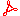FLOC 2022: FEDERATED LOGIC CONFERENCE 2022
POS POS'22 FLOC PAPERS: PAPERS WITH ABSTRACTS

Editors: Daniel Le Berre and Matti Järvisalo

Authors, Title and AbstractPaperTalk

ABSTRACT. SAT-solvers are one of the primary tools to assess the security of block ciphers automatically. Common CNF encodings of s-boxes are based on algebraic representations (finding low-degree polynomials) or symbolic execution of considered function implementation. However, those methods are not strictly connected with algorithms used in efficient SAT-solvers. Therefore, we propose an application of minimal propagate complete encodings, which in their definition are tuned for modern satisfiability checking algorithms.

During the construction of the Boolean formula, there is often the problem of encoding linear XOR equations. The standard procedure includes a greedy shortening algorithm to decrease the size of the resulting encoding. Recently, the problem of a straight-line program has been successfully applied in obtaining efficient implementations of MDS matrices. In this paper, we propose to use the algorithm for finding a short straight-line program as a shortening procedure for a system of linear XOR equations.

As a result, we achieved a 20x speed-up of algebraic cryptanalysis of Small Scale AES block cipher to widely used algebraic representations by combining two approaches.Aug 01 09:00

ABSTRACT. In this paper we report our preliminary results with a new kind of SAT solver called Dinosat. Dinosat's input is a conjunction of clauses, at-most-one constraints and disjunctive normal form (DNF) formulas. The native support for DNF formulas is motivated by the application domain of SAT based product configuration. A DNF formula can also be viewed as a generalization of a clause, i.e., a clause (disjunction of literals) is special case of a DNF formula, where each term (conjunction of literals) has exactly one literal. Similarly, we can generalize the classical resolution rule and use it to resolve two DNF formulas. Based on that, the CDCL algorithm can be modified to work with DNF formulas instead of just clauses. Using randomly generated formulas (with DNFs) we experimentally show, that in certain relevant scenarios, it is more efficient to solve these formulas with Dinosat than translate them to CNF and use a state-of-the-art SAT solver. Another contribution of this paper is identifying the phase transition points for such formulas.Aug 01 11:00

ABSTRACT. In this study, we propose a new framework for easily constructing efficient deterministic parallel SAT solvers, providing the delayed clause exchange technique introduced in ManyGlucose. This framework allows existing sequential SAT solvers to be parallelized with just as little effort as in the non-deterministic parallel solver framework such as PaInleSS. We show experimentally that parallel solvers built using this framework have performance comparable to state-of-the-art non-deterministic parallel SAT solvers while ensuring reproducible behavior.Aug 01 11:30

ABSTRACT. We give a first account of our new parallel SAT solver Gimsatul. Its key feature is to share clauses physically in memory instead of copying them, which is the method of other state-of-the-art multi-threaded SAT solvers to share clauses logically. Our approach keeps information about which literals are watched in a clause local to a solving thread but shares the actual immutable literals of a clause globally among all solving threads. This design gives quiet remarkable parallel scalability, allows aggressive clause sharing while keeping memory usage low and produces more compact proofs.Aug 01 12:00

ABSTRACT. Traditional Boolean satisfiability (SAT) solvers based on the conflict-driven clause-learning (CDCL) framework fare poorly on formulas involving large numbers of parity constraints. The CryptoMiniSat solver augments CDCL with Gauss-Jordan elimination to greatly improve performance on these formulas. Integrating the TBUDDY proof-generating BDD library into CryptoMiniSat enables it to generate unsatisfiability proofs when using Gauss-Jordan elimination. These proofs are compatible with standard, clausal proof frameworks.Aug 01 14:30

ABSTRACT. The Pigeonhole Principle (PHP) has been heavily studied in automated reasoning, both theoretically and in practice. Most solvers have exponential runtime and proof length, while some specialized techniques achieve polynomial runtime and proof length. Several decades ago, Cook manually constructed O(n^4) extended resolution proofs, where n denotes the number of pigeons. Existing automated techniques only surpass Cook's proofs in similar proof systems for large n. We construct the shortest known proofs of PHP in the standard proof format of modern SAT solving, DRAT. Using auxiliary variables and by recursively decomposing the original program into smaller sizes, we manually obtain proofs having length O(n^3) and leading coefficient 5/2.Aug 01 15:00

ABSTRACT. Visual layouts of graphs representing SAT instances can highlight the community structure of SAT instances. The community structure of SAT instances has been associated with both instance hardness and known clause quality heuristics. Our tool SATViz visualizes CNF formulas using the variable interaction graph and a force-directed layout algorithm. With SATViz, clause proofs can be animated to continuously highlight variables that occur in a moving window of recently learned clauses. If needed, SATViz can also create new layouts of the variable interaction graph with the adjusted edge weights. In this paper, we describe the structure and feature set of SATViz. We also present some interesting visualizations created with SATViz.Aug 01 16:00